Next: 5.2.4 Integrated Color Maps Up: 5.2 Images Previous: 5.2.2 Galactic and Extragalactic   Contents   Index

## 5.2.3 Extragalactic velocity field

In this example we shall make a velocity field of a a particle representation of a disk galaxy, with stars on circular orbits in centrifugal balance with a fixed background potential.

For disk-stars on circular orbits the program mkdisk is useful5.9:

   21% mkdisk out=disk1 potname=expdisk potpars=0,1,0.5 rmax=2 mass=1


In this the mass of the disk was set to non-zero, in order to assign a finite emission to each star later on. If you would plot it's configuration with a program like snapplot, you would see not only a more-or-less constant surface density but also that the disk is infinitely thin (snapplot yvar=z). Also the particles are indeed on circular orbits (snapplot xvar=r yvar=vt), and there is no velocity dispersion (snapplot xvar=r yvar=vr).

Viewed from the positive Z axis (the default with snapplot xvar=x yvar=y) we would see no radial velocities in the disk; in order to get a realistic looking velocity field, we would have to rotate the model around a line of nodes (say the X axis) using snaprotate:

    22% snaprotate in=disk1 out=disk1.r theta=60 order=x


To plot the radial velocity the program snapplot is used by assigning a different symbol (psize=) to different radial velocities:

    23% snapplot in=disk1.r psize=0.1*vz


To simulate a true observation we shall use the program snapgrid to grid the discrete snapshot data (x,y,z,vx,vy,vz) from the file disk1.r onto a CCD-like device: a rectangular pixel array (matrix), with a value (brightness, velocity etc.) associated with each pixel. Since we are interested in the radial velocity field the zero-th and first order moment maps need to be obtained, and divided to get a radial velocity field: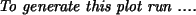Here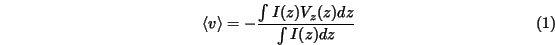and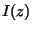are the intensity and radial velocity along the line of sight. Note the extra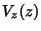sign, to conform to the astronomical convention that positive velocity means negative vz if viewed from the positive Z axis. In NEMO the denominator and numerator in eq. (1) are evaluated as follows:

    24% snapgrid in=disk1.r out=mom0 moment=0 zvar=-vz evar=m
25% snapgrid in=disk1.r out=mom1 moment=1 zvar=-vz evar=m


Since the data will be noisy, it is best to smooth the data a bit. Smoothing must however be done before the maps are divided (why?). Since the default pixel size is 4/64=0.0625 a Gaussian beam with a FWHM of 0.15 is used to convolve the data. We would use the programs ccdsmooth and ccdmath in the following order:

   26% ccdsmooth in=mom0 out=mom0s gauss=0.15
27% ccdsmooth in=mom1 out=mom1s gauss=0.15
28% ccdmath in=mom0s,mom1s out=disk1.vel fie=%2/%1


The final output file, disk1.vel, now contains the radial velocity field map at an inclination of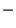. It can be displayed with programs like ccdplot and ds. ccdplot is a NEMO program, capable of plotting contours as well as greyscale (if given the right graphics device driver). ds is general purpose image display program and displays a map in color on a sun workstation (for this, ds must have been installed to understand the NEMO file format).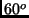You can also convert the NEMO image file to a FITS file. A FITS file is a true astronomical standard, which can be read into any other image processing package (ds can also read FITS files) (AIPS, IRAF, MIDAS, Miriad). Creating it can be done as follows:

    29% ccdfits in=velfie out=fits1Next: 5.2.4 Integrated Color Maps Up: 5.2 Images Previous: 5.2.2 Galactic and Extragalactic   Contents   Index
(c) Peter Teuben